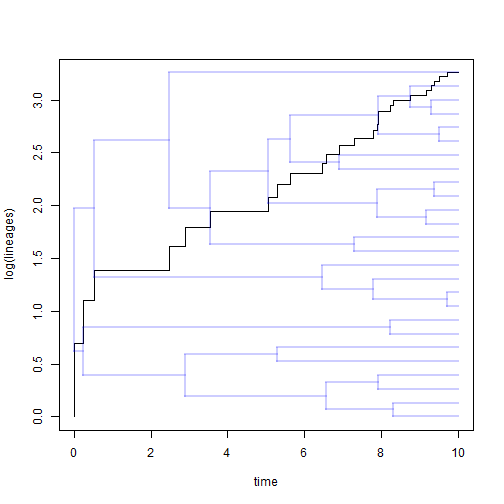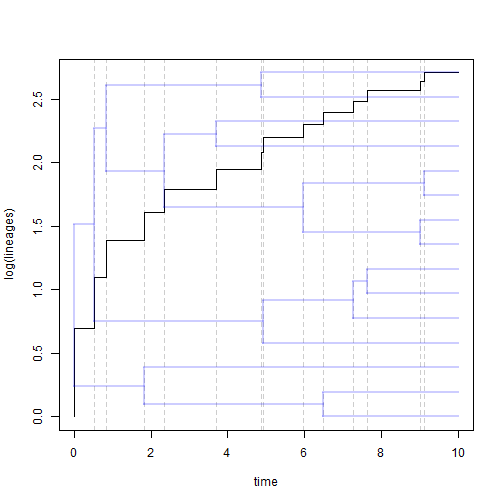## Friday, May 29, 2015

### Overlaying a tree on an LTT plot

I'm just in the middle of fixing a bug in the phytools function for lineage-through-time plots, `ltt`, pointed out to me by Luke Harmon. It has to do with node labels & is not that interesting (but I will nonetheless post the fixed version soon). However, in the process of playing around with that function, a discovered a find of neat visualization that involves overlaying a phylogeny on an LTT plot.

Here is a demo:

``````library(phytools)
## simulate tree
tree<-pbtree(n=26,scale=10) ## arbitrary
``````

The next part uses a function to automatically generate from a starting color a color with a certain `alpha` level of transparency. This function was posted to R-sig-phylo by Josef Uyeda:

``````makeTransparent<-function(someColor,alpha=10){
newColor<-col2rgb(someColor)
apply(newColor,2,function(curcoldata){
rgb(red=curcoldata,green=curcoldata,blue=curcoldata,
alpha=alpha,maxColorValue=255)
})
}
``````

Now we're ready to create our visualization:

``````obj<-ltt(tree)
mar=par()\$mar)
``````We might even add other features - like vertical lines so we can see where lineages that have accumulated appear. This works well particularly for small trees:

``````tree<-pbtree(n=15,scale=10)
obj<-ltt(tree)
for(i in 3:(length(obj\$ltt)-1))
lines(rep(obj\$times[i],2),par()\$usr[3:4],lty="dashed",
col=makeTransparent("black",alpha=50))